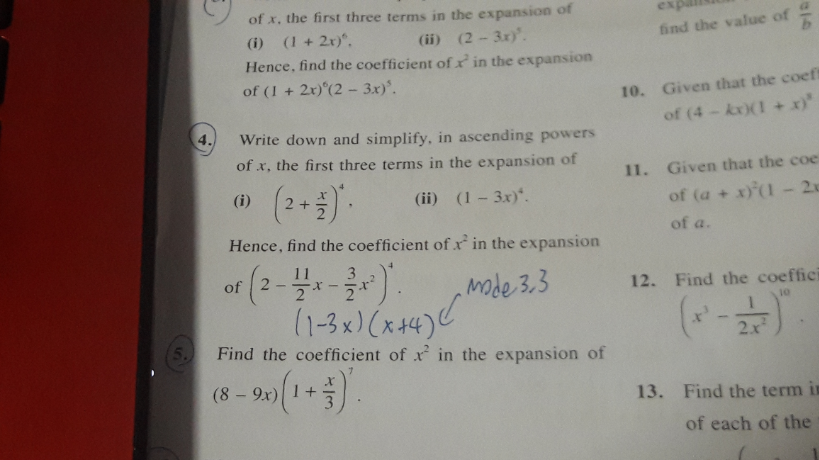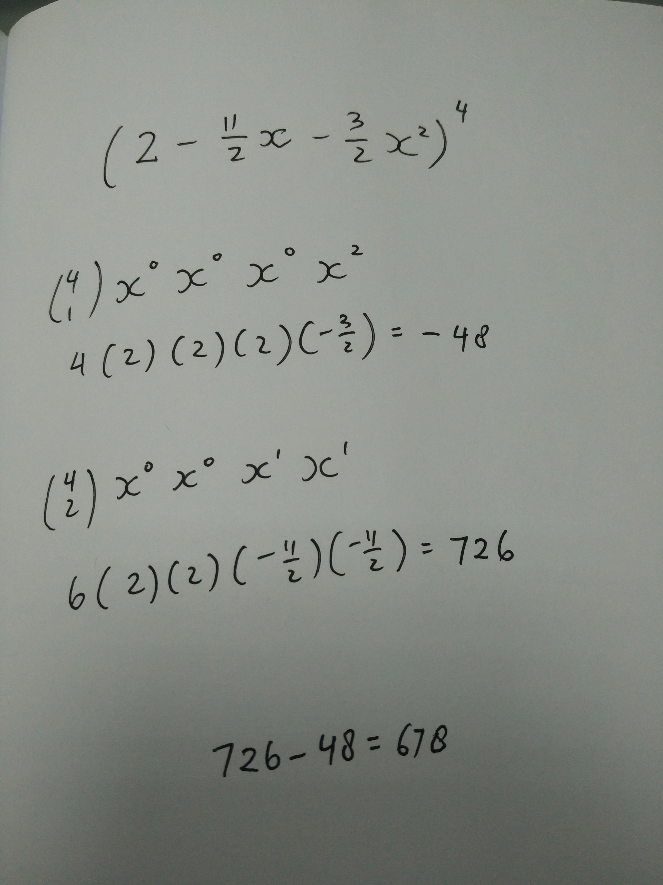QuestionI’m looking for the solution to question 4, or more specifically the factorisation of (2-11/2x-3/2x^2)^4You may want to consider this method as it is more straightforward

interesting solution.

Can explain a bit more?

Are you expanding it as

(2+(-11/2x-3/2x^2))^4

sorry for the late…

and yeah i expanded it as it is… i think i shouldn’t since it is a “HENCE” question

0 Replies 0 Likes

( 2 – 11/2 x – 3/2 x² )

= [ ( 2 + x/2 ) ( 1 – 3x ) ]4

=  ( 2 + x/2 )4 ( 1 – 3x )4

= ( 16 + 16x + 6x² +… ) ( 1 – 12x + 54x² +…)

the coefficient of x² in the expansion of  ( 2 – 11/2 x – 3/2 x² )

= 16*54 + 16*(-12) + 6*1

= 678

Hi jieheng, could you clarify how (2-11/2 x -3/2x^2) became (2 + x/2)(1-3x)? If you used cross method, how do you use it with terms that are fractions?

clearly (1-3x)(x+4) is not equal to 2-11/2 x -1.5×2.

after using mode 3, 3 to get the roots of the quad eq, you have to check that yr factorisation is correct. There are many ways of writing a quad expression with the same roots.

For such binomial questions, i would suggest you to expand the expression in part (i) and part (ii) and see how it is related to the later binomial expansion.  In this question, when you expand (1-3x)(2+x/2), you will get 2-11/2x -3×2/2. Then you can proceed with your solution.

If this is a emaths question on factorisation (2-11/2 x -3/2 x2), you can do in as follow:

2-11/2x -3/2×2 =-1/2(3×2+11x-4)=-1/2(x+4)(3x-1).

ps…hope you can understand. Do not know how to type the power using hp.